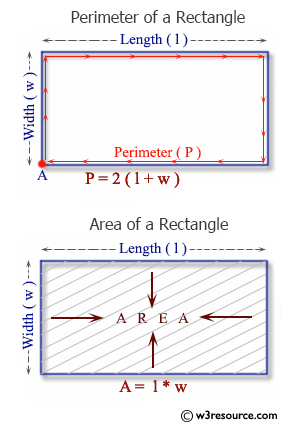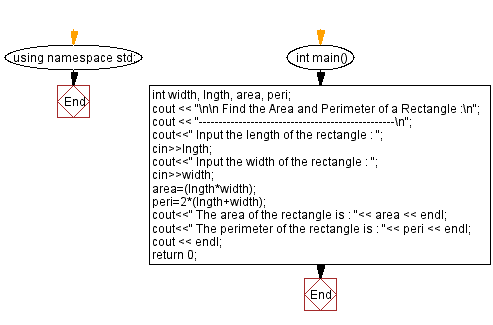﻿ C++ Exercises: Find the Area and Perimeter of a Rectangle - w3resource

# C++ Exercises: Find the Area and Perimeter of a Rectangle

## C++ Basic: Exercise-17 with Solution

Write a C++ program to find the Area and Perimeter of a Rectangle.

Pictorial Presentation:Sample Solution:

C++ Code :

``````#include <iostream>
using namespace std;

int main()
{
int width, lngth, area, peri;
cout << "\n\n Find the Area and Perimeter of a Rectangle :\n";
cout << "-------------------------------------------------\n";
cout<<" Input the length of the rectangle : ";
cin>>lngth;
cout<<" Input the width of the rectangle : ";
cin>>width;
area=(lngth*width);
peri=2*(lngth+width);
cout<<" The area of the rectangle is : "<< area << endl;
cout<<" The perimeter of the rectangle is : "<< peri << endl;
cout << endl;
return 0;
}
``````

Sample Output:

``` Find the Area and Perimeter of a Rectangle :
-------------------------------------------------
Input the length of the rectangle : 10
Input the width of the rectangle : 15
The area of the rectangle is : 150
The perimeter of the rectangle is : 50
```

Flowchart:C++ Code Editor:

What is the difficulty level of this exercise?

﻿

## C++ Programming: Tips of the Day

Why is there no std::stou?

The most pat answer would be that the C library has no corresponding "strtou", and the C++11 string functions are all just thinly veiled wrappers around the C library functions: The std::sto* functions mirror strto*, and the std::to_string functions use sprintf.

Ref: https://bit.ly/3wtz2qA

We are closing our Disqus commenting system for some maintenanace issues. You may write to us at reach[at]yahoo[dot]com or visit us at Facebook